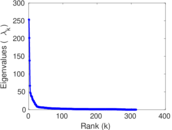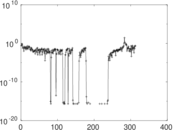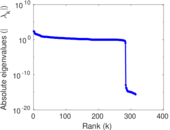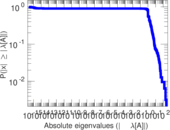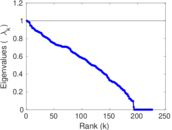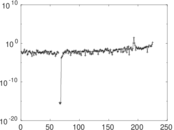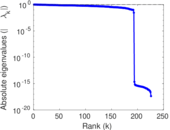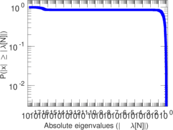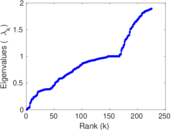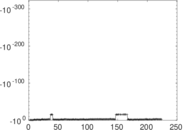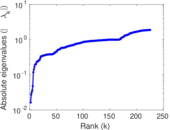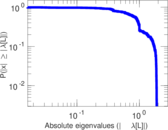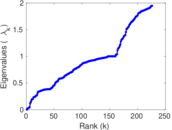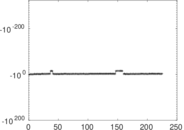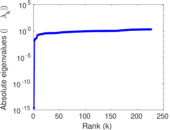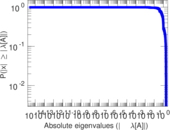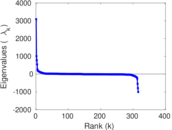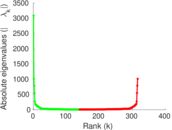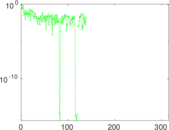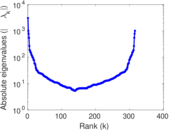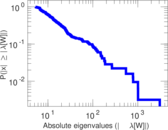# Wikiquote edits (ta)

This is the bipartite edit network of the Tamil Wikiquote. It contains users and pages from the Tamil Wikiquote, connected by edit events. Each edge represents an edit. The dataset includes the timestamp of each edit.

 Code `qta` Internal name `edit-tawikiquote` Name Wikiquote edits (ta) Data source http://dumps.wikimedia.org/ AvailabilityDataset is available for download Consistency checkDataset passed all tests Category Authorship network Dataset timestamp 2017-10-20 Node meaning User, article Edge meaning Edit Network formatBipartite, undirected Edge typeUnweighted, multiple edges Temporal dataEdges are annotated with timestamps

## Statistics

 Size n = 3,097 Left size n1 = 316 Right size n2 = 2,781 Volume m = 9,857 Unique edge count m̿ = 4,305 Wedge count s = 703,865 Claw count z = 132,751,728 Cross count x = 21,065,027,363 Square count q = 46,649 4-Tour count T4 = 3,197,486 Maximum degree dmax = 2,448 Maximum left degree d1max = 2,448 Maximum right degree d2max = 602 Average degree d = 6.365 52 Average left degree d1 = 31.193 0 Average right degree d2 = 3.544 41 Fill p = 0.004 898 75 Average edge multiplicity m̃ = 2.289 66 Size of LCC N = 2,844 Diameter δ = 13 50-Percentile effective diameter δ0.5 = 3.505 17 90-Percentile effective diameter δ0.9 = 5.724 00 Median distance δM = 4 Mean distance δm = 4.160 11 Gini coefficient G = 0.770 955 Balanced inequality ratio P = 0.191 184 Left balanced inequality ratio P1 = 0.106 625 Right balanced inequality ratio P2 = 0.259 815 Relative edge distribution entropy Her = 0.783 274 Power law exponent γ = 4.120 95 Tail power law exponent γt = 2.411 00 Tail power law exponent with p γ3 = 2.411 00 p-value p = 0.000 00 Left tail power law exponent with p γ3,1 = 1.781 00 Left p-value p1 = 0.648 000 Right tail power law exponent with p γ3,2 = 2.621 00 Right p-value p2 = 0.000 00 Degree assortativity ρ = −0.292 921 Degree assortativity p-value pρ = 6.235 84 × 10−86 Spectral norm α = 253.156 Algebraic connectivity a = 0.016 205 9 Spectral separation |λ1[A] / λ2[A]| = 1.255 27 Controllability C = 2,521 Relative controllability Cr = 0.816 650

## Plots

### Fruchterman–Reingold graph drawing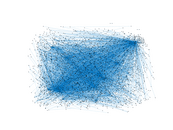### Degree distribution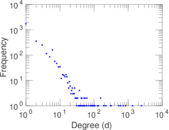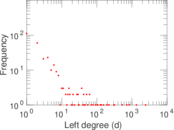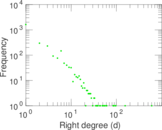### Cumulative degree distribution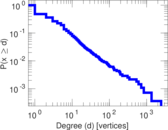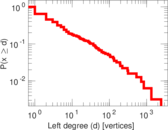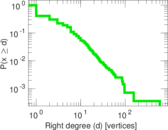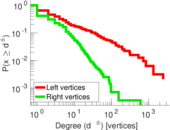### Lorenz curve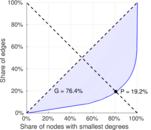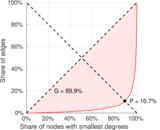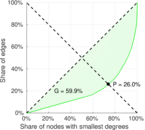### Spectral distribution of the adjacency matrix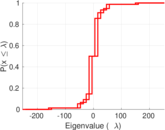### Spectral distribution of the normalized adjacency matrix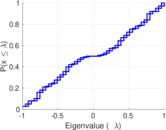### Spectral distribution of the Laplacian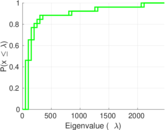### Spectral graph drawing based on the adjacency matrix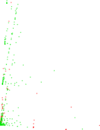### Spectral graph drawing based on the Laplacian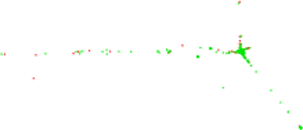### Spectral graph drawing based on the normalized adjacency matrix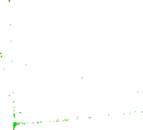### Degree assortativity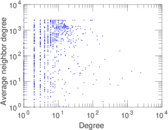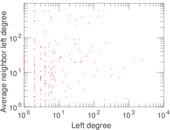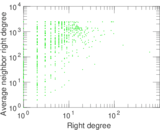### Zipf plot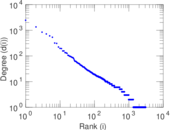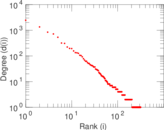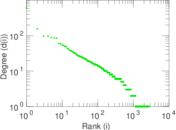### Hop distribution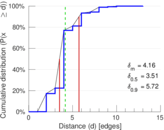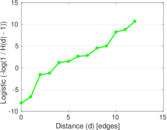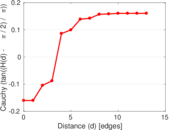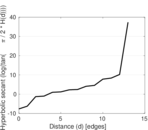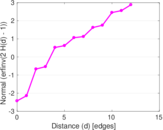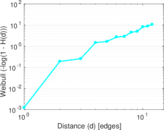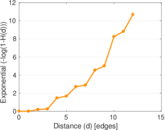### Double Laplacian graph drawing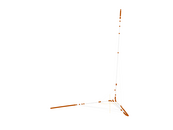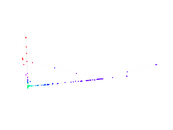### Delaunay graph drawing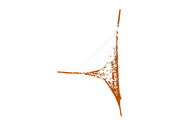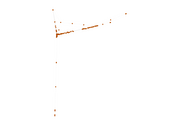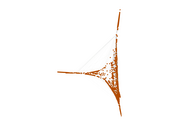### Edge weight/multiplicity distribution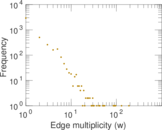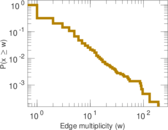### Temporal distribution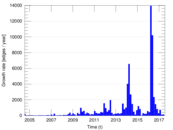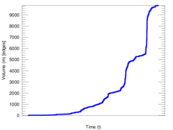### Temporal hop distribution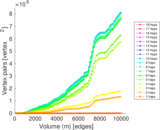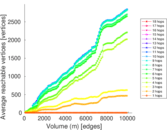### Diameter/density evolution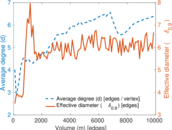### Matrix decompositions plots